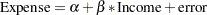# Example: Survey Sampling and Analysis Procedures

This section demonstrates how you can use the survey procedures to select a probability-based sample and then analyze the survey data to make inferences about the population. The analyses include descriptive statistics and regression analysis. This example is a survey of income and expenditures for a group of households in North Carolina and South Carolina. The goals of the survey are as follows:

• Estimate total income and total living expenses

• Estimate the median income and the median living expenses

• Investigate the linear relationship between income and living expenses

### Sample Selection

To select a sample with PROC SURVEYSELECT, you input a SAS data set that contains the sampling frame (the list of units from which the sample is to be selected). You also specify the selection method, the desired sample size or sampling rate, and other selection parameters. PROC SURVEYSELECT selects the sample and produces an output data set that contains the selected units, their selection probabilities, and their sampling weights. See Chapter 91, The SURVEYSELECT Procedure, for more information about PROC SURVEYSELECT.

In this example, the sample design is a stratified sample design, with households as the sampling units and selection by simple random sampling. The SAS data set HHFrame contains the sampling frame, which is the list of households in the survey population. The sampling frame is stratified by the variables State and Region. Within strata, households are selected by simple random sampling. The following PROC SURVEYSELECT statements select a probability sample of households according to this sample design:

```   proc surveyselect data=HHFrame out=HHSample
method=srs n=(3, 5, 3, 6, 2);
strata State Region;
run;
```

The STRATA statement names the stratification variables State and Region. In the PROC SURVEYSELECT statement, the DATA= option names the SAS data set HHFrame as the input data set (or sampling frame) from which to select the sample. The OUT= option stores the sample in the SAS data set named HHSample. The METHOD=SRS option specifies simple random sampling as the sample selection method. The N= option specifies the stratum sample sizes.

The SURVEYSELECT procedure then selects a stratified random sample of households and produces the output data set HHSample, which contains the selected households together with their selection probabilities and sampling weights. The data set HHSample also contains the sampling unit identification variable Id and the stratification variables State and Region from the input data set HHFrame.

### Survey Data Analysis

You can use the SURVEYMEANS and SURVEYREG procedures to estimate population values and perform regression analyses for survey data. The following example briefly shows the capabilities of these procedures. See Chapter 88, The SURVEYMEANS Procedure, and Chapter 90, The SURVEYREG Procedure, for more information.

The following PROC SURVEYMEANS statements estimate the total income and living expenses for the survey population based on the data from the stratified sample design:

```   proc surveymeans data=HHSample sum median;
var Income Expense;
strata State Region;
weight Weight;
run;
```

The PROC SURVEYMEANS statement invokes the procedure, and the DATA= option names the SAS data set HHSample as the input data set to be analyzed. The keywords SUM and MEDIAN request estimates of population totals and medians.

The VAR statement specifies the two analysis variables Income and Expense. The STRATA statement names the stratification variables State and Region. The WEIGHT statement specifies the sampling weight variable Weight.

You can use PROC SURVEYREG to perform regression analysis for survey data. Suppose that, in order to explore the relationship between household income and living expenses in the survey population, you choose the following linear model:The following PROC SURVEYREG statements fit this linear model for the survey population based on the data from the stratified sample design:

```   proc surveyreg data=HHSample;
strata State Region ;
model  Expense = Income;
weight Weight;
run;
```

The STRATA statement names the stratification variables State and Region. The MODEL statement specifies the model, with Expense as the dependent variable and Income as the independent variable. The WEIGHT statement specifies the sampling weight variable Weight.